# Synthesis of nickel ferrite nanoparticles as an efficient magnetic sorbent for removal of an azo-dye: Response surface methodology and neural network modeling

Document Type : Research Paper

Authors

1 Department of Chemistry, Faculty of Sciences, Azarbaijan Shahid Madani University, Tabriz, Iran

2 Department of Chemistry, Payame Noor University, Tehran, Iran

Abstract

In this research, nickel ferrite (NiFe2O4) nanoparticles (NFNs) are prepared through coprecipitation method, and applied for adsorption removal of a model organic pollutant, methyl orange (MO). The characterization of the prepared NFNs was performed using scanning electron microscopy (SEM), X-ray diffraction (XRD), vibrating sample magnetometer (VSM) and transmission electron microscopy (TEM). Optimization and modeling of the removal of MO applying NFNs were performed via central composite design (CCD) and the influential parameters including nano-sorbent amount, dye initial concentration, contact time and pH were considered as input variables for CCD. A dye removal percentage of 99 % was achieved under the optimum condition established for MO removal that was in agreeing with the predicted value. Additionally, multi-layer artificial neural network (ML-ANN) was applied to acquire a predictive model of MO removal. The isothermal investigation of MO adsorption was performed by developing Langmuir, Freundlich and Temkin models, and results showed that experimental data were best fit in Freundlich model. Based on the adsorption kinetics studies, the pseudo-second-order kinetic model was the best model to describe the adsorption mechanism of MO onto NFNs.

Keywords

#### Full Text

INTRODUCTION

Nowadays, pollution of water resources due to the various pollutants is an important global issue. Extensive usage of dyes in different industries leads to release of large amount of these pollutants in the environment leading to create many hazardous problems in environment [1, 2]. Usually, colors persist against the conventional removal processes and cause the difficulties in treatment of water contamination . Many of these dyes are carcinogenic, mutagenic, and teratogenic and also toxic to human beings, fish species, and microorganisms. Hence, their removal from aquatic wastewater becomes environmentally important .

Methyl orange (MO) is one of the famous anionic and acidic azo dyes which is extensively utilized in textile, printing, paper, pharmaceutical and food industries . Because of the low biodegradability of MO and the presence of azo (N=N) group in MO chemical structure, its removal from wastewaters and industrial effluents is important issue in order to decrease its hazardous effect on the environment. Among various physical and chemical water treatment procedures applied for treatment of wastewater, adsorptive removal is widely used because of its specific properties such as non-toxic, versatility of adsorbents, high adsorption capacity and high removal percentage for available sorbents, being inexpensive, adsorbent regenerability in addition to slug free process .

In recent years, nanomaterials with their unique chemical and physical properties, exhibiting high surface areas and high surface activity along with various surface atoms have shown effective interactions with various chemicals. Among the different classes of nanomaterials, the application of magnetic nanoparticles is a promising way for adsorption process [7-9]. The application of magnetic adsorbents causes to introduce a new field in separation methods considering their unique properties. When a magnetic field with low intensity is applied to the magnetic nanoparticles, they are magnetized and therefore, are separated by applying a magnetic force. Various spinel magnetic nanoparticles with general formula (AB2O4) such as NiFe2O4, MnFe2O4, CoFe2O4 and MgFe2O4 have been applied as magnetic adsorbents [10-13]. While there are numerous studies documented on nickel ferrite as a magnetic, catalytic and gas sensing material, studies on adsorption properties of nickel ferrite are very limited [14, 15]. Most of the various magnetic nanoparticles have the drawbacks of adsorption low capacity and especially inefficient regeneration of the adsorbents, which lead to their limited practical application. Nickel ferrite is one of the most versatile important magnetic materials due to its high saturation magnetization, low conductivity high Curie temperature, high chemical and electrochemical stability, catalytic behavior, and relatively high permeability that make nickel ferrite an efficient and capable adsorbent [16, 17].

In this research, nickel ferrite nanoparticles are synthesized through coprecipitation of nickel nitrate and ferric nitrate in an alkaline media. The capability of NFNs for the adsorption of MO is investigated. The removal efficiency is modeled and optimized concerning the effects of the initial concentration of MO, pH, adsorbent amount and contact time applying central composite design. The model obtained and significances of various parameters are evaluated by analysis of variance (ANOVA). Moreover, multilayer feed-forward neural network model is treated and used to predict the removal of MO in the adsorption process. Finally, the MO adsorption isotherms and kinetic study are performed.

EXPERIMENTS

Materials

Chemicals of nickel nitrate tetrahydrate (Ni(NO3)2·۴H2O), ferric nitrate hexahydrate (Fe(NO3)3·۶H2O), nitric acid, NH4HCO3, NH3, sodium hydroxide and hydrochloric acid were all supplied by Merck (Germany). An acidic dye, methyl orange (MO) (C14H14N3NaO3S, M.W: 327.33 g mol-1), was used as a model compound which was provided by Merck (the structure of MO is presented in Fig. 1).

Instruments

The concentration of MO was determined using a Shimadzo UV-Vis spectrophotometer (Tokyo, Japan) at λmax = 503 nm. In order to adjust solution pH, a Metrohm 827 pH/Ion meter (Switzerland) was applied. A shaker (Sahand.T.a., Iran) was applied to agitate sample during adsorptive removal process.

The magnetic separation of NFNs was performed using a permanent magnet of Nd-Fe-B (8.0 cm × 6.0 cm × 1.6 cm). The characterization of prepared NFNs was carried out using transmission electron microscopy (TEM) (Philips, Netherland), X-ray diffraction (Philips PW1800 instrument, Netherland) and vibrating sample magnetometer (VSM) using MDKFD instrument (Iran).

Preparation of nickel ferrite nanoprticles

Nickel ferrite nanaoparticles have been synthesized according to the previously documented literature . Briefly, 14.4 g of Ni(NO3)2 6H2O was dissolved in 10 mL water. Then, 26.9 g Fe(NO3)3 6H2O dissolved in 10 mL water was added to the firstly prepared solution. The resulted solution was then agitated at 50 ºC for 1 h. Next, the solution of NH3 was added slowly with stirring. The gel formed was dried in an oven with temperature of 40 ºC for 72 h to get precursor. Finally, the dried precursor was calcinated at 350 ºC for 1 h to obtain pure NiFe2O4.

Batch removal experiments

In order to evaluate the capability of prepared nickel ferrite nanoparticles for adsorption of MO, batch adsorption experiments were performed in 50 mL of dye solution with different concentrations. The solution pH was adjusted by adding HCl 1 M or NaOH 1 M solution and a pH meter. The predetermined amount of NFNs was added to the MO solution and adsorptive removal process was conducted by agitation of the solutions on a shaker. After a desired contact time, the NFNs were magnetically separated by applying an external magnet (8.0 cm × 6.0 cm × 1.6 cm) to the bottom of the beaker and supernatant was removed to determine the concentration of remaining MO by spectrophotometric determination at λmax value of 503 nm. Finally, the dye removal percentages (R %) were calculated as follows: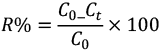(1)

where C0 (mg L-1) is initial dye concentration and Ct (mg L-1) is related to the dye concentration after time t. For studying the adsorption kinetic and adsorption isotherms, the equilibrium adsorption capacity (qe, mg g-1) and adsorption capacity at certain time (qt, mg g-1) were obtained using the following equations (Eqs (2) and (3)):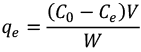(2)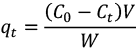(3)

where V (L) denotes the solution volume and W (g) is the adsorbent mass.

Design of experiments

Central composite design which is commonly used in response surface modeling was applied to model and to optimize the MO adsorptive removal process. Four factors influencing the removal percentage (k = 4) have been considered as input variables for CCD, including pH, initial concentration of MO, adsorbent amount and contact time that their actual and coded values are reported in Table 1. The removal percentage (R %) was regarded as a response of model. 31 experiments were designed with four input variables for studying the removal process consisting of 16 (2k) orthogonal full factorial design (coded at level of ±1), 8 (2k) star points (coded as ±α = 2.0) and 7 replications in the central point to estimate the experimental error variance. Minintab 16 software was employed to design the experiments and analysis of experimental data. The significance and adequacy of the model obtained were evaluated by ANOVA. The correlation coefficient, R2 was applied to judge statistically the quality of the regression equation, and F-test was used to evaluate the significance of model. The confidence level of 95%, and therefore, P-value of 0.05 was considered to evaluate the statistically significance of model and models terms.

Definition of the ANN model

Artificial neural network is a flexible mathematical structure which is able to determine nonlinear relationships between independent variables and output data. Among various ANN structures, the multilayer feed forward neural network is extensively used structure to achieve nonlinear regression models. The typical ANN constructed by three different layers including an input layer, one or more hidden layers and an output layer. According to the universal approximation theory, for an ANN with less output than inputs, one hidden layer is enough , therefore in this work, a multilayer feed-forward ANN consisting one hidden layer was used using sigmoid transfer function for the hidden layer and linear transfer function for the output node. In order to train the ANN, Levenberg-Marquardt back-propagation algorithm was used with 100 iterations. All calculations and modeling were performed using Matlab 2011 mathematical software with the ANN toolbox. The ANN inputs are pH, NFNs amount, exposure time and dye concentration, while dye removal percentage was considered as the output. Input data were obtained from CCD, and, in order to avoid numerical overflows, because of very large or small weights, all inputs and outputs were normalized within a uniform range of (0.1 to 0.9) according to the Eq. (4):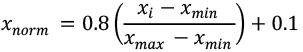(4)

where xnorm is related to the normalized value of xi, xmax and xmin denote the maximum and minimum values of xi, respectively.

The experimental data were divided into two groups (24 experiments based on CCD except of replicates on central point for training and 13 more experiments for testing set). In order to select the optimum structure for ANN, the mean square error (MSE) of the test data along with the correlation coefficient (R2) were employed as criteria, which can be expressed as follows: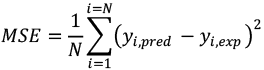(5)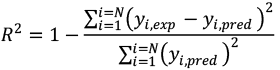(6)

where yi,exp and yi,pred are the desired and calculated outputs, respectively.

RESULTS AND DISCUSSION

Characterization of nickel ferrite nanoparticles

Characterization of nickel ferrite nanocrystallites was performed using XRD (Fig. 2), TEM and SEM (Fig. 3). XRD spectrum of the prepared sample was investigated in order to confirm the formation of magnetic NFNs in the synthesized sample. The XRD pattern of the NFNs (Fig. 2) illustrates that these nanoparticles have spinel structure due to the presence of the most intense peak (311). In addition, Miller indices (400), (422), (440) and (511) are well matched with the XRD spectrum of the references nickel ferrite sample . The average crystallite size of the prepared NFNs was calculated according to the Scherrer equation: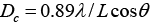(7)

where Dc denotes the crystallite diameter, θ is the diffraction angle of the peak, λ is the X-ray wavelength, and L is related to the full width at half maximum intensity (FWHM) of the diffraction peak. The average crystallite size of the prepared NFNs was obtained to be 15 nm, accordingly.

The SEM and TEM micrograph of the sample is displayed in Fig. 3. As can be seen in TEM image, it is demonstrated that the prepared magnetic nanoparticles are spherical, well dispersed and narrowly size distributed with average size of 10 to 15 nm in the diameter.

Magnetic property of the prepared NFNs was investigated using vibrating sample magnetometer (VSM) at room temperature. The applied magnetic field was in the range of -9000 to 9000 Oe and the parameters of coercive field (Hc) and saturation magnetization (Ms) were investigated. Coercivity, also known as coercive force, is defined as the force needed for removing the residual magnetism from the material. Fig. 4. shows VSM plot of NFNs at 300 K. As can be seen, magnetic remanence is not observed in the hysteresis loop, indicating that the nanoparticles can be considered as super-paramagnetic material (Hc=0 Oe). The magnetization saturation (Ms) of the NFNs was 3.93 emu g-1. According to the obtained results, the prepared nickel ferrite can be considered as a super-paramagnetic material without coercivety. Moreover, it has appropriate magnetic characteristic leading to efficiently separation of nanoparticles from aquatic solutions.

Development of RSM model

Generally, RSM is a capable statistical tool which uses quantitative data obtained from well-designed experiments to achieve a multivariate equation, and its main aim is obtaining optimum value for the studied variables. Usually, a second-order polynomial model also known as regression equation, (Eq. (8)), is used to model the response of the systems as a function of main effects, two factor interaction effects and curvature effects of the studied factors. This regression equation describes an approximation of experimental results at various experimental conditions according to the following equation: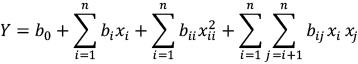(8)

where Y is the response of the system, xi is the coded levels of the factors, b0 is a constant and bi, bii and bij are the main effect, curvature effect and interaction effects for the corresponding factors, respectively, which are obtained using ordinary least square [21, 22].

Based on the analysis of experimental data obtained using designed experiments, the second-order regression equation considering the coded levels of factors for describing the MO removal percentage using NFNs-based nano-sorbent can be written as the following equation (Eq. (9)):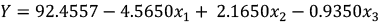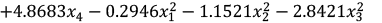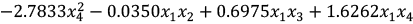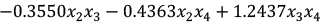(9)

where, x1, x2, x3 and x4 are dye concentration, exposure time, pH and amount of adsorbent, respectively. In order to evaluate the quality and significance of the obtained model, analysis of variance was conducted and the obtained data are tabulated in Table 2. Accordingly, F-value corresponding to regression is 6.54 which is higher than the tabulated F-value (2.352 at significance of 95%). In addition, regression P-value is 0.000 and less than 0.05 (at the confidence level of 95%) indicating the statistically significance of regression model. Moreover, the lack of fit (LOF) test is carried out as another criterion by comparing the residuals with experimental pure error, leading to lower F-value than tabulated value and greater P value than 0.05, at the significance level of 95 %, (0.058), demonstrating the insignificant lack of fit and adequacy of the model.

Additionally, for evaluating the significance of the developed model, the residuals can be used to study the model significance graphically. When the regression model would be a good descriptor of response as a function of variables, residuals should be occurred in a normal distribution manner . The plot of normal probability versus internally studentized residuals is displayed in Fig. 5a. As can be seen, the normal probability plot is a relatively straight line and no significant dispersal of the experimental data is observed, indicating the adequacy of the model. Moreover, the plot of residuals versus fitted dye removal (%) in Fig. 5b and the plot of residuals versus run number in Fig. 5d illustrate the random dispersal of residuals, confirming a good fitness between the predicted responses and experimental data. Furthermore, according to the histogram of residuals depicted in Fig. 5c, rather normal distribution of residuals is indicated.

Effect of variables on the MO removal efficiency

The effectiveness and significance of each term in the obtained model were determined considering the corresponding Student’s t values and P-values (Table 3). The greater T-value and smaller P-value (less than 0.05 at significance level of 95%) for a coefficient indicate the important effect of the coefficient [24, 25]. Considering the data presented in Table 3, the insignificant coefficients with P-value greater than 0.05 in Eq. (9) were omitted and the mentioned equation was rewritten as Eq. (10).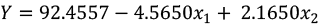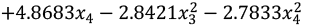(10)

As demonstrated in Eq. (10), the regression model for MO removal percentage consists three main effects, and two curvature effects without any interaction effect. Furthermore, the effect of each factor on the MO removal efficiency was determined by calculating the corresponding percentage effect according to Pareto analysis (Eq. (11)) :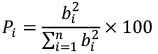(11)

where Pi is the each term percentage effect and bi is coefficient of each factor. According to the results, displayed in the Fig. 6, the main effect of sorbent amount (b4, 32.93%) is the most effective term. After that, the main effect of dye concentration (b1, 28.96%), the main effect of contact time (b2, 6.51 %), the curvature effect of pH (b33, 11.22 %) and the curvature effect of sorbent dosage (b44, 10.76%) are important. Sorbent amount is one of the important factors with positive sign, indicating the increase of removal efficiency with increase of sorbent dosage (Fig. 7c, 7e and 7f). Application of the greater sorbent amount causes the availability of the more adsorption sites and surface area, and consequently the higher removal percentage for the desired pollutant.

Another important parameter influencing the removal efficiency is dye initial concentration that is significant with negative sign. The effect of this factor on the MO removal efficiency is depicted on the Pareto analysis and also response surface plot (Fig. 6, 7a, 7b and 7c). As can be seen, dye concentration exhibits a negative effect on removal percentage and its increasing leads to the decrease of response. The lower dye removal percentage at higher initial dye concentrations is might be due to the saturation of adsorptive sites on the surface of NF nanoparticles.

As resulted, the curvature effect of pH is important in MO removal efficiency. The results revealed that the removal percentage of MO is reduced at acidic and alkaline media. It is worth to be noted that the isoelectric point of NFNs is 7 and at pH values lower than this point, the surface of NFNs is positively charged and an electrostatic attraction can be established between sorbent surface and anionic target molecule. Also, the reduction of removal percentage is reasonable as the surface of NFNs is negatively charged which has a repulsion force to the anionic MO. At pH lower than 5.5, MO may undergo the protonation reaction and attractive forces between MO and NFNs can be diminished.

Numerical optimization

The final goal of CCD is numerical optimization of the studied factors performed by setting the values of independent parameters in the studied range and setting the model response (dye removal) in maximum value. According to the obtained results, when adsorbent dosage of 70 mg, initial dye concentration of 50 mg L-1, contact time of 31.5 min, and pH of 5.5 were applied the maximum dye removal of 99 % was obtained. In order to approve the obtained results, confirmatory experiment was conducted using the optimum practical condition. Accordingly, a dye removal percentage of 99 % was obtained using optimum condition, indicating the accuracy and capability of the developed model for predicting dye removal at various experimental conditions.

Alternatively, considering the q value as the system response, the optimum values for the studied parameters leading to the maximum value for q were as follows: adsorbent amount of 25 mg, initial dye concentration of 300 mg L-1, contact time of 60 min and pH of 4.4. Considering this set of experimental condition, the predicted value for q was 148 mg g-1 which has been confirmed by evaluating q value at the as mentioned optimum condition.

ANN modeling

In this research, multilayer feed-forward ANN with one hidden layer was applied. For all data sets, sigmoid transfer function was applied for the hidden layer and a linear transfer function was used for the output layer. The input variables for feed-forward ANN were the contact time, dye concentration, pH and sorbent amount. The MO removal percentage was the experimental response or output variable. The topology of an ANN is defined using the nature of the transfer functions, the number of layers and the number of nodes in each layer. In order to build ANN model, the first task is correct selecting the independent input variables and the dependent output variables. For developing the ANN model, the next step is the optimization of ANN topology. In this regard, various numbers of nodes (N) in the hidden layer were examined and the results were evaluated considering the prediction error of the ANN which should be a minimum value. The prediction error is an important parameter for the evaluation of neural network prediction capability, therefore it is usually considered for this purpose .

For determining the optimum numbers of node in hidden layer, different topologies for ANN were treated, in which the number of neurons was changed between 2 to 18. Fig. 8 depicts the mean error of examined networks versus the node numbers in the hidden layer. Accordingly, the presence of five nodes in the hidden layer has caused the lower mean square error (MSE) for the trained neural network. Therefore, a three-layer feed-forward back propagation neural network with five nodes in the hidden layer was applied to model the adsorptive removal process (Fig. 9). The weights provided by ANN are presented in Tables 4 and 5. In order to examine the modeled ANN, an independent set of data (test set) was used and the predicted output values were compared with the experimental results. The plot of the experimental results versus the predicted values is displayed in Fig. 10, demonstrating that the points construct the line of with a correlation coefficient of R2 = 0.965, indicating the reliability of the model.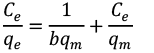(12)

where Ce is the concentration of non-adsorbed target species in solution at the equilibrium (mg L-1) and qe the amount of adsorbed species per gram of adsorbent at equilibrium (mg g-1). The Langmuir constants, qm (mg g-1), is related to the capacity of monolayer adsorption and b (L mg-1) denotes the adsorption heat.

The Freundlich isotherm is suitable to describe the multilayer adsorption with heterogeneous surface energies and can be presented in a linear form as follows: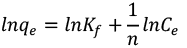(13)

where Kf (mg g-1) indicates the approximate adsorption capacity , and 1/n represents the intensity of adsorption and also the favorability of adsorption. The value higher than one represents the favorable adsorption condition . .

According to the Temkin isotherm equation, the heat of adsorption of all the adsorbates in a layer decreases linearly with surface coverage because of adsorbate-adsorbent interactions. Moreover, it is assumed that there is a uniform distribution of the bonding energies, up to some maximum binding energy for the adsorption process. The Temkin isotherm is presented in linear form as Eq. (14):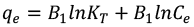(14)

where B1 =RT/b is related to the adsorption heat, T and R denote the absolute temperature in Kelvin and the universal gas constant (8.314 J mol-1 K-1), respectively . KT is the equilibrium binding constant (L mol-1) related to the maximum binding energy. A plot of qe versus lnCe leads to determine the isotherm constants KT and B1 from the intercept and the slope, respectively.

In this work, the discussed isotherm models for describing the adsorption of MO onto NFNs were investigated by exposing 70 mg of the nanosorbent to the 50 ml solutions containing various concentrations of MO in the range of 50–350 mg L-1 at pH= 5.5. After 31.5 min, the concentration of remained MO in the solution was determined and the obtained results were applied to construct the isotherm models using Eqs. (12) to (14). The calculated parameters for adsorption isotherms are presented in detailed in Table 6. Based on the results, experimental data fit almost well in Freundlich isotherm model, suggesting multilayer adsorption of MO onto the nickel ferrite nanoparticles with heterogeneous surface energies.

The kinetic study of adsorption process provides helpful information about adsorption rate and the efficiency of adsorption. Hence, pseudo-first-order , second order , pseudo-second-order , Elovich [36, 37] and intraparticle diffusion [36, 38]ate¸¦| F­ kinetic models were studied for adsorption of MO onto nickel ferrite nanoparticles. The obtained correlation coefficients for various kinetic models were used to evaluate the consistency between the experimental and predicted data.

In order to evaluate the kinetic aspects of MO adsorption, 70 mg of the NFNs was exposed to MO in 50 mL solution at concentration level of 50 mg L-1 at pH= 5.5 and room temperature. In order to determine the well fitted kinetic model, the Lagergren pseudo-first-order, the pseudo-second-order, and the second-order models according to Eqs. (15-17) were examined: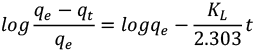(15)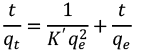(16)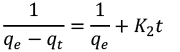(17)

where KL (min-1), K´ (g mg-1 min-1) and K2 (g mg-1 min-1) are the rate constants for Lagergren pseudo-first-order model, pseudo-second-order model, and second-order model, respectively. qt and qe are the amounts of solute adsorbed (mg g-1) at time t and at equilibrium, respectively.

The Elovich kinetic model is another rate equation based on the adsorption capacity that is given as follows (Eq. (18)) :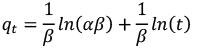(18)

where, α (mg g-1min-1) and β (g mg-1) are initial adsorption rate and desorption constant, respectively. Plotting qt versus ln(t) will give a straight line with a slope of (1/β) and an intercept of (1/β) ln(αβ) if the experimental data are well fitted in Elovich model. The Elovich constants resulted from the intercept and the slope of the linear relationship are tabulated in Table 7.

Another alternative model to study the kinetic of an adsorption process is intra-particle-diffusion . Based on this model, transport of target species from aqueous solution to the adsorbents takes place by intra-particle diffusion according to the following equation (Eq. (19)):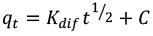(19)

Plot of qt versus t1/2 yields a linear relationship in which the values of Kdif and C are calculated from the slopes; the corresponding values are presented in Table 7. There are two linear parts in plot of qt versus t1/2, and the rate constant Kdif is directly obtained from the slope of the second regression line. When the value of C is zero intra-particle diffusion is the only rate-limiting step, indicating that the intra-particle diffusion model may be the main factor in the kinetic of the adsorption process . The closer R2 values (Table 7) to unity suggests the applicability of this kinetic model and that the intra-particle diffusion process is not the rate-limiting step.

Based on the developed kinetics models for adsorption of MO onto nickel ferrite nanoparticles using the experimental data, the corresponding kinetic parameters and correlation coefficients (R2) were obtained and presented in Table 7. The best kinetic model was selected based on the highest correlation coefficient (R2), that is related to the pseudo second-order adsorption model. Additionally, the value of qe(cal) calculated by pseudo second-order model is very close to that of the experimental value, qe(exp) in Table 7. Accordingly, the pseudo second-order model is predominant for describing the MO adsorption mechanism onto NFNs.

Finally, the adsorptive performance of synthesized NFNs for MO removal has been compared with performance of activated carbon/Fe3O4 nanocomposite; the data are presented in Table 8. Accordingly, both adsorption methods follow pseudo-second order kinetics model. Adsorption of MO on the NFNs follows Freundlich isotherm while its adsorption on the activated carbon/Fe3O4nanocomposite follows both Freundlich and Langmuir isotherms. The adsorbent capacity for the NFNs was 148 mg g-1 while for activated carbon/Fe3O4 was 182 mg g-1. The equilibrium time for the NFNs was 60 min that is less than equilibrium time for activated carbon/Fe3O4 (200 min). Accordingly, it can be concluded that the prepared NFNs can be regarded as efficient and capable adsorbent for MO removal from aquatic media.

CONCLUDING REMARKS

The synthesis of nickel ferrite nanoparticles was carried out by chemical coprecipitation of nickel nitrate and ferric nitrate in the alkaline media. The application of prepared nanoparticles as an adsorbent was investigated for the removal of MO as an anionic azo dye model compound from aqueous solutions. The effects of adsorbent amount, pH, contact time and initial dye concentration on the MO removal percentage were investigated using CCD. A dye removal percentage of 99 % was obtained under the established optimum condition for MO removal, in agreeing with the predicted value. Considering the q value as the response of the system and applying experimental optimum condition, q was 148 mg g-1. A three-layer ANN (using a back-propagation algorithm) with four, five and one nodes in input, hidden and output layers, respectively, was treated and successfully applied to predict the performance of removal process of MO. For all data sets, sigmoid transfer function was used in the hidden layer and a linear transfer function was used for output layer. The isothermal investigation of MO adsorption was performed by developing Langmuir, Freundlich and Temkin models, and the results showed that experimental data fit well in Freundlich model. On the basis of adsorption kinetics studies, the pseudo-second-order kinetic model was the best model to describe the adsorption mechanism of the MO onto NFNs. Finally, the prepared magnetic nanosorbent was characterized by a considerable high stability, high adsorption capability and magnetically separation capability, making it a suitable product for MO removal as model compound from solutions.

COMPLIANCE WITH ETHICAL STANDARDS

Funding: The financial support of this work was provided by the Research Councils of Payame Noor University (Tehran) and Azarbaijan Shahid Madani University.

Conflict of Interest: Zahra Ayazi declares that she has no conflict of interest. Zahra Monsef Khoshhesab declares that she has no conflict of interest. Alireza Amani-Ghadim declares that he has no conflict of interest.

Ethical Approval: This article does not contain any studies with human participants or animals performed by any of the authors.

Informed Consent: Not applicable.